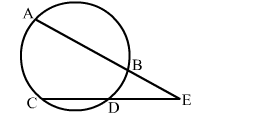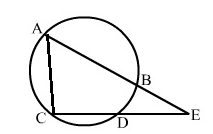# Two chords AB and CD of a circle intersect each other at a point E outside the circle.

Question:

Two chords AB and CD of a circle intersect each other at a point E outside the circle. If AB = 11 cm, BE = 3 cm and DE = 3.5 cm, then CD = ?
(a) 10.5 cm
(b) 9.5 cm
(c) 8.5 cm
(d) 7.5 cmSolution:

(c) 8.5 cm
Join AC.Then AE : CE = DE : BE     (Intersecting secant theorem)
∴ AE × BE = DE × CE
Let CD = x cm
Then AE = (AB + BE) = (11 + 3) cm = 14 cm; BE = 3cm; CE = (x + 3.5) cm; DE = 3.5 cm
∴ 14 × 3 = (x + 3.5) × 3.5

$\Rightarrow x+3.5=\frac{14 \times 3}{3.5}=\frac{42}{3.5}=12$

⇒ x = (12 - 3.5) cm = 8.5 cm
Hence, CD = 8.5 cm